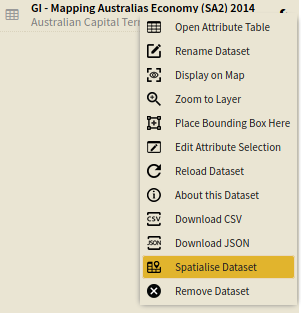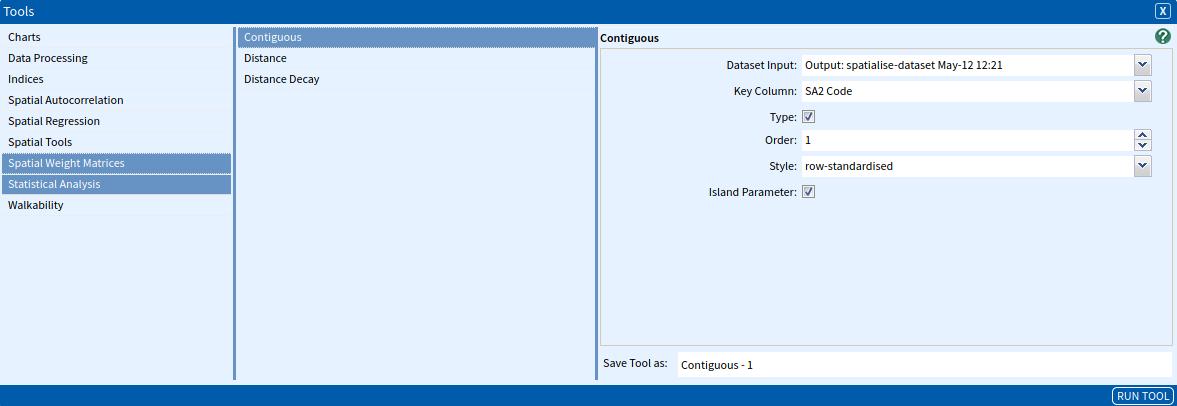Contiguous Spatial Weight Matrix

The Contiguous Spatial Weight Matrix tool quantifies the spatial relationships between features in a dataset, where features are considered contiguous if they share a common boundary.

Weights are expressed in an $n \times n$ matrix $\mathbf{W}$ where each element $w_{ij}$ is a spatial weight.

$\mathbf{W} =\begin{bmatrix} w_{11} & w_{12} & \cdots & w_{1n}\\ w_{21} & w_{22} & \cdots & w_{2n}\\ \vdots & \vdots & \ddots & \vdots\\ w_{n1} & w_{n2} & \cdots & w_{nn} \end{bmatrix}$

The spatial weights $w_{ij}$ are non-zero when $i$ and $j$ are contiguous neighbours, and zero otherwise. While this is more straightforward than other methods for determining spatial weight matrices, there are additional levels of complexity.

Rook and Queen Contiguity

There are two different means by which a feature could be contiguous with its neighbours. Rook contiguity defines neighbours as two polygons that share a common boundary. Queen contiguity defines neighbours as two polygons that share a common boundary or vertex.

This is illustrated in the image below for mesh-blocks in Canberra. For the rook contiguity case (dark red), the neighbouring mesh-blocks are shown in light red. For the queen contiguity case (dark blue), the neighbouring mesh-block are shown in light blue.Order of Contiguity

In addition to determining how contiguity is defined in a directional sense, we can also determine how far we consider a neighbouring polygon to be. This is known as the order of contiguity. The images above represent 1st order contiguity, where the immediately adjacent mesh-blocks appear within the spatial weight matrix as contiguous to the mesh-block in question. By comparison, 2nd order contiguity counts those polygons contiguous with the polygon in question (1st order contiguous polygons), as well as those contiguous with the 1st order polygons.

The 2nd order contiguity representation of the Canberra mesh-blocks is illustrated in the image below, where the lightest red or blue mesh-blocks represent the 2nd order contiguity mesh-blocks for the rook and queen contiguity methods, respectively.SET UP

To illustrate this tool, we will use economic data for the Australian Capital Territory.

Select Australian Capital Territory GCCSA as your area.

Select GI – Mapping Australias Economy (SA2) 2014 as your dataset, selecting all variables.

Spatialise your dataset by using the Spatialise Aggregated Dataset tool or by selecting Spatialise Dataset from the spanner menu (see below).Inputs

Once you have loaded and spatialised your dataset, navigate to the Contiguous Spatial Weight Matrix tool (Tools → Spatial Weight Matrices → Contiguous) and enter the following parameters:

• Dataset Input: The dataset that you would like to calculate the spatial weights matrix – this must be spatialised for the tool to run (either by using the Spatialise Aggregate Dataset tool with and AURIN dataset or by uploading a geospatial file). Select the Spatialised Dataset.
• Key column: The unique identifier for each row. Select SA2 Code.
• Type: This specifies whether queen contiguity (ticked) or rook contiguity (unticked). Tick the checkbox to select Queen.
• Order: This specifies how many orders of contiguity. Select 1 for 1st order of contiguity.
• Style: This specifies whether the standardisation will be row-standardised, binary or globally-standardised. It is standard practice to standardise these matrices so that locations that are contiguous with a large number of neighbours not over-emphasised within the analysis. Select row-standardised.
• row-standardised: Creates proportional weights by dividing each neighbour weight for a feature by the row total.
• binary: Assigns a value of 1 to contiguous features and a 0 to all other features.
• globally-standardised: Creates proportional weights by dividing each neighbour weight by the global total.
• Island Parameter: If ticked, the tool allows the matrix to be computed with no-neighbour features, if unticked, the presence of no-neighbour features issues an error message. Tick the checkbox.

The input parameters are summarised in the image below, once complete click Run Tool.Outputs

Once the tool has run, open the output dataset and it should look like the image below.Elements with zero scores indicate that the features are not contiguous, and therefore do not affect each other. If a value is greater than zero, it indicates that the area specified by the column influences the area of the row to the extent of the value. It is important to realise that the values along the row will be identical – that is, each area that is contiguous with the target area influences it equally (even in 2nd or more order contiguity).

Looking for Spatial Data?

You can browse the AURIN Data Discovery:How can you Create Impact?